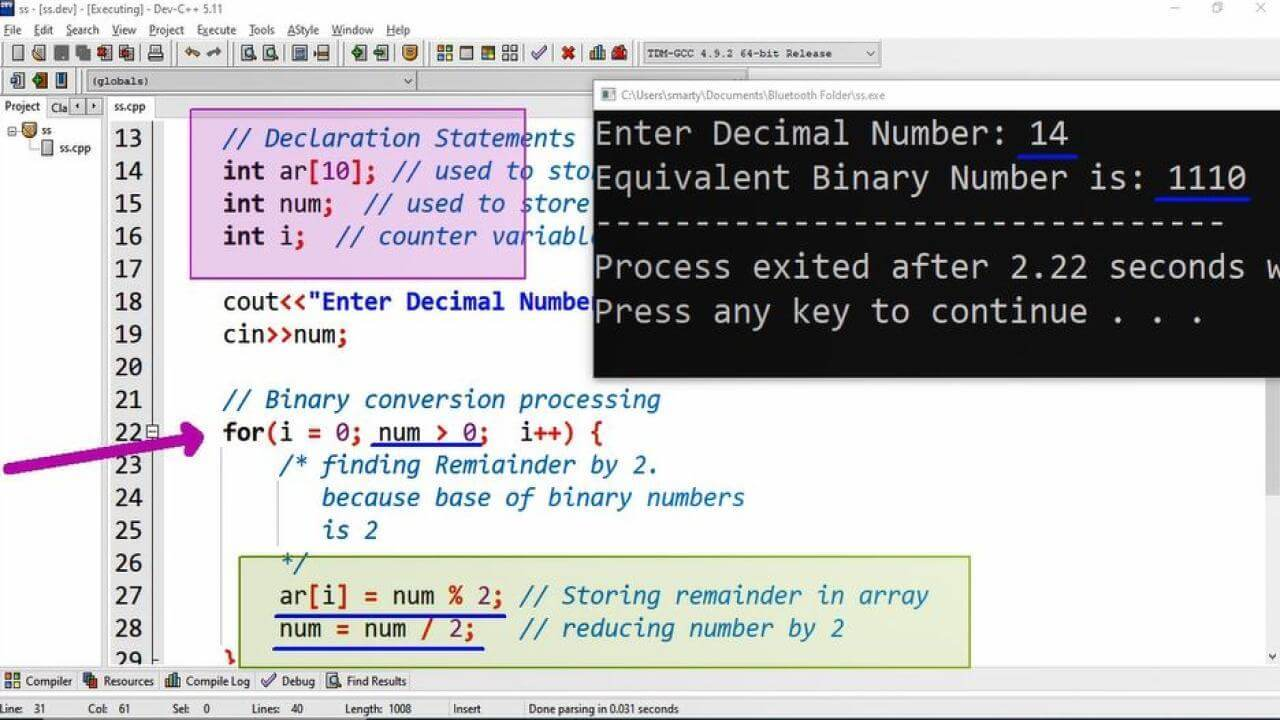# Decimal To Binary Conversion Program Example using CPP

## Source Code:

#include <iostream>
using namespace std;

/*
Decimal To Binary is converted Using Remainder Method.

*/

int main(int argc, char** argv) {

// Declaration Statements
int ar; // used to store binary digits
int num;  // used to store decimal input
int i;  // counter variable

cout<<"Enter Decimal Number: ";
cin>>num;

// Binary conversion processing
for(i = 0; num > 0;  i++) {
/* finding Remiainder by 2.
because base of binary numbers
is 2
*/

ar[i] = num % 2; // Storing remainder in array
num = num / 2;   // reducing number by 2
}

i--;  // returning counter variable to last digit index.
//printing equivalent binary number
cout<<"Equivalent Binary Number is: ";
while( i >= 0) { // loop will iterate until first index
cout<<ar[i];   // displaying array elements
i--;  // printing array from right to left.
}

return 0;
}

## Output:## Working:

In this program example we are going to convert a decimal number taken by user as input. To get equivalent binary number we have to repeat the following steps.

1. Take remainder of given number
2. Put this remainder in successive array index
3. Divide number by 2.
4. Repeat from Step 1

To complete the process of conversion first of all we declare an array of size 10. The size of array may be diffeent according to the required processing of decimal number. If we want to convert large decimal numbers then we will have to increase the size of array.

The list of variables used in this program is as provided.

 Sr Identifier Type Description 1 ar int This array will store the binary digits 2 num int num variable is used to take decimal input from the user. 3 i int i is counter variable used in loop